Next: Dimensions and entropies Up: Lyapunov exponents Previous: The maximal exponent

## The Lyapunov spectrum

The computation of the full Lyapunov spectrum requires considerably more effort than just the maximal exponent. An essential ingredient is some estimate of the local Jacobians, i.e. of the linearized dynamics, which rules the growth of infinitesimal perturbations. One either finds it from direct fits of local linear models of the type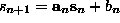, such that the first row of the Jacobian is the vector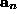, and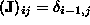for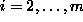, where m is the embedding dimension. Theis given by the least squares minimization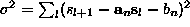where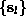is the set of neighbors of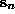[45, 71]. Or one constructs a global nonlinear model and computes its local Jacobians by taking derivatives. In both cases, one multiplies the Jacobians one by one, following the trajectory, to as many different vectors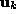in tangent space as one wants to compute Lyapunov exponents. Every few steps, one applies a Gram-Schmidt orthonormalization procedure to the set of, and accumulates the logarithms of their rescaling factors. Their average, in the order of the Gram-Schmidt procedure, give the Lyapunov exponents in descending order. The routine lyap_spec uses this method, which goes back to  and , employing local linear fits. Apart from the problem of spurious exponents, this method contains some other pitfalls: It assumes that there exist well defined Jacobians, and does not test for their relevance. In particular, when attractors are thin in the embedding space, some (or all) of the local Jacobians might be estimated very badly. Then the whole product can suffer from these bad estimates and the exponents are correspondingly wrong. Thus the global nonlinear approach can be superior, if a modeling has been successful, see Sec..

In Tablewe show the exponents of the stroboscopic NMR laser data in a three dimensional embedding as a function of the neighborhood size. Using global nonlinear models, we find the numbers given in the last two rows. More material is discussed in . The spread of values in the table for this rather clean data set reflects the difficulty of estimating Lyapunov spectra from time series, which has to be done with great care. In particular, when the algorithm is blindly applied to data from a random process, it cannot internally check for the consistency of the assumption of an underlying dynamical system. Therefore a Lyapunov spectrum is computed which now is completely meaningless.

 method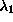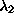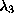local linear k=20 0.32 -0.40 -1.13 `` k=40 0.30 -0.51 -1.21 `` k=160 0.28 -0.68 -1.31 radial basis functions 0.27 -0.64 -1.31 polynomial 0.27 -0.64 -1.15

Table:   Lyapunov exponents of the NMR laser data, determined with a three-dimensional embedding. The algorithms described in Sec.give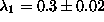for the largest exponent.

The computation of the first part of the Lyapunov spectrum allows for some interesting cross-checks. It was conjectured , and is found to be correct in most physical situations, that the Lyapunov spectrum and the fractal dimension of an attractor are closely related. If the expanding and least contracting directions in space are continuously filled and only one partial dimension is fractal, then one can ask for the dimensionality of a (fractal) volume such that it is invariant, i.e. such that the sum of the corresponding Lyapunov exponents vanishes, where the last one is weighted with the non-integer part of the dimension: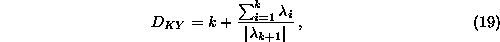where k is the maximum integer such that the sum of the k largest exponents is still non-negative.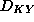is conjectured to coincide with the information dimension.

The Pesin identity is valid under the same assumptions and allows to compute the KS-entropy: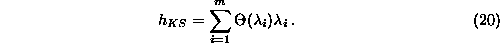Next: Dimensions and entropies Up: Lyapunov exponents Previous: The maximal exponent

Thomas Schreiber
Wed Jan 6 15:38:27 CET 1999